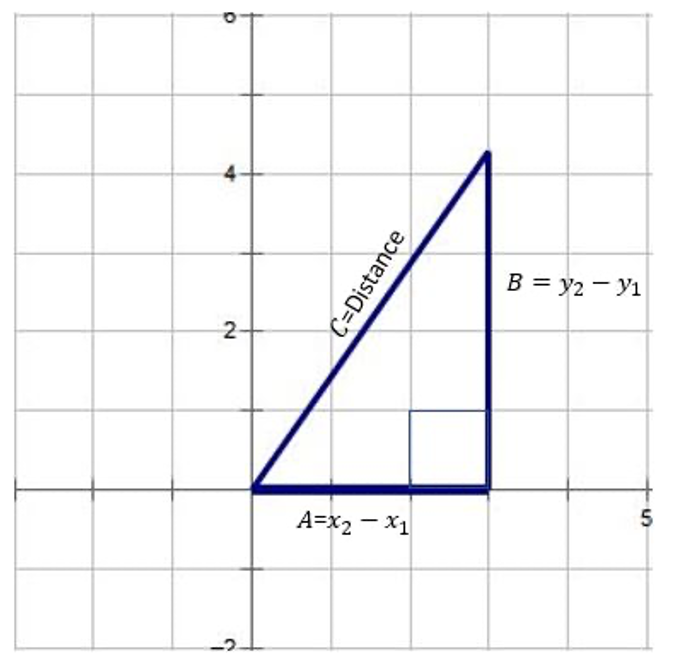# What is the distance formula? A variation of the Pythagorean Theorem

The distance formula is used to find the distance between any two points on the coordinate plane. The distance formula is a variation of the Pythagorean Theorem.## What is the Distance Formula?

The distance formula is used to find the distance between any two points on the coordinate plane.

### The Distance Formula

The distance between two points

is given by

Does the distance formula look familiar to you at all?It should be, because the distance formula is actually a variation of the Pythagorean Theorem! Recall, that the Pythagorean Theorem is

and can be used to find a missing side of a right triangle.

### How does the Distance Formula work?

To show how the Pythagorean Theorem can be transformed into the distance formula, we first want to take our right triangle onto the coordinate plane.

Next, we will change our side lengths to represent distance instead. For example, the length of A in the image above is 3 units long on the x-axis. We can represent this in distance form by taking the largest x-value of the triangle and subtracting it from the smallest x-value of the triangle. The largest value (X2) and the smallest value (X1) is 0. Therefore, the distance of the length of A can be written as (3-0) or (X2-X1). This can be also done using the length of B, but the general distance will be (Y2-Y1).

Finally, we can use the Pythagorean theorem to find the distance of the triangle above.

### Distance Formula Problem Example 1:

Find the distance between the points (-27, 4) and (19,-6).

#### Step 2: Substitute the values in the formula and simplify.

Thus, the distance between (-27, 4) and (19, -6) is47.07 units long.

### Distance Formula Problem Example 2:

Find the distance between the points

#### Step 2: Substitute the values in the formula and simplify.

Thus, the distance between (20,3) and (15,5) is 5.38 units long.

#### MORE MATH PROBLEMS# Need more help? Get Algebra or Geometry Tutoring

Remind Tutoring provides 1 on 1 online math tutoring. Your experienced tutor can help you work through difficult problems and help you master algebra, geometry, and other math concepts. What is the Distance formula? How do you use the Pythagorean theorem? No problem. The first session is free, and it's \$40 per session after that. If you need some help in Algebra or Geometry or any other math, get a math tutor to help.